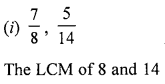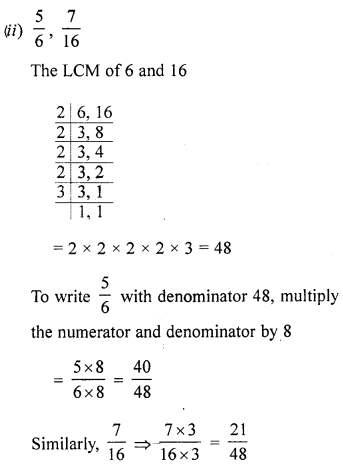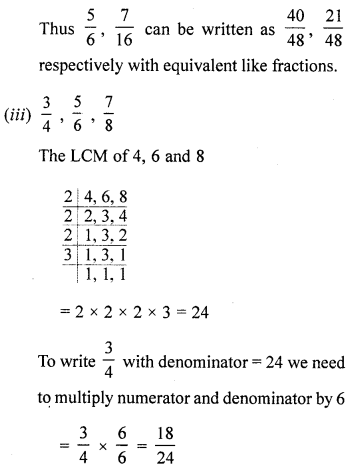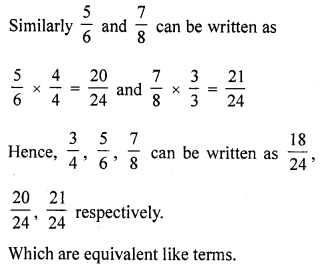## ML Aggarwal Class 6 Solutions for ICSE Maths Chapter 6 Fractions Ex 6.3

Question 1.
State which of the following fractions are proper, improper or mixed: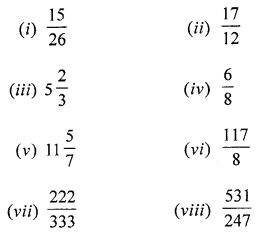Solution: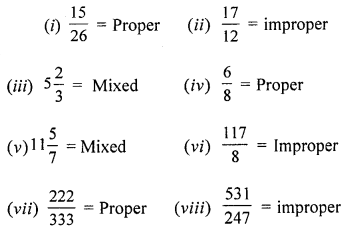Question 2.
Convert the following improper fractions into mixed numbers: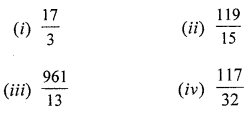Solution: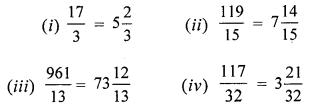Question 3.
Convert the following mixed number into improper fractions: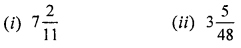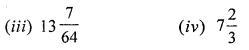Solution: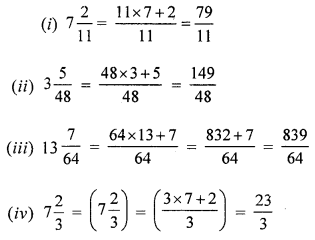Question 4.
Write the fractions representing the shaded regions. Are all these fractions equivalent?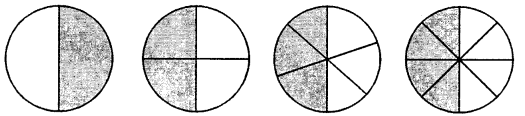Solution:Yes, all the fractions are equivalent.

Question 5.
Write the fractions representing the shaded regions and pair up the equivalent fractions from each row: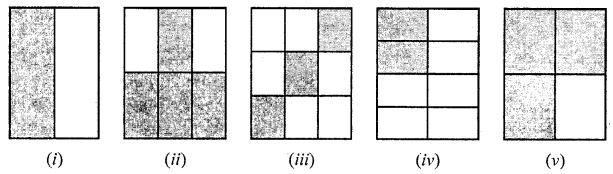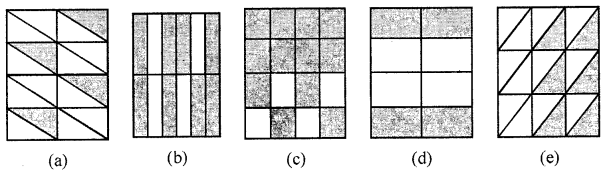Solution:Equivalent fractions are:
(i) ↔ (d)
(ii) ↔ (b)
(iii) ↔ (e)
(iv) ↔ (a)
(v) ↔ (c)

Question 6.
(i) Find the equivalent fraction of $$\frac{15}{35}$$ with denominator 7.
(ii) Find the equivalent fraction of $$\frac{2}{9}$$ with denominator 63.
Solution:
(i) $$\frac{15}{35}=\frac{ . . .}{7}$$
Let the numerator be a
⇒ 15 × 7 = 35 × a
$$a=\frac{15 \times 7}{35}$$
⇒ a = 3
∴ $$\frac{15}{35}=\frac{3}{7}$$

(ii) $$\frac{2}{9}=\frac{\dots}{63}$$
Let the numerator, which needs to be calculated as x
⇒ 2 × 63 = 9 × x
⇒ $$x=\frac{2 \times 63}{9}$$
⇒ x = 14
∴ $$\frac{2}{9}=\frac{14}{63}$$

Question 7.
Find the equivalent fraction of $$\frac{3}{5}$$ having
(i) denominator 30
(ii) numerator 27.
Solution:
(i) $$\frac{3}{5}$$ having denominator 30
Multiply and divide the fraction by 6, we get
$$\frac{3}{5} \times \frac{6}{6}=\frac{18}{30}$$

(ii) $$\frac{3}{5}$$ having numerator 27
Multiply and divide the fraction by 9, we get
$$\frac{3}{5} \times \frac{9}{9}=\frac{27}{45}$$

Question 8.
Replace ‘…..’ in each of the following by the correct number.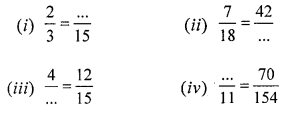Solution: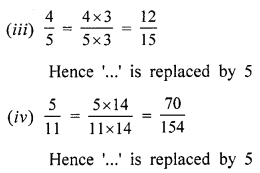Question 9.
Check whether the given pairs of fractions are equivalent:Solution:Question 10.
Reduce the following fractions to simplest form: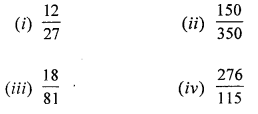Solution: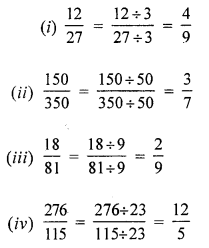Question 11.
Convert the following fractions into equivalent like fractions:Solution: